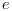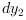Scilab Home page | Wiki | Bug tracker | Forge | Mailing list archives | ATOMS | File exchange
Change language to: English - Português - 日本語 - Русский

Please note that the recommended version of Scilab is 6.0.2. This page might be outdated.
See the recommended documentation of this function

lqg

LQG compensator

Syntax

K=lqg(P_aug,r)
K=lqg(P,Qxu,Qwv)
K=lqg(P,Qxu,Qwv,Qi,#dof)

Arguments

P_aug

State space representation of the augmented plant (see: lqg2stan)

r

1 by 2 row vector = (number of measurements, number of inputs) (dimension of the 2,2 part of P_aug)

P

State-space representation of the nominal plant (nu inputs, ny outputs, nx states).

Qxu

Symmetric nx+nu by nx+nu weighting matrix.

Qwv

Symmetric nx+ny by nx+ny covariance matrix.

Qi

Symmetric ny by ny weight for integral term.

#dof

Scalar with value in {1,2}, the degrees of freedom of the controller. The default value is 2.

K

Linear LQG (H2) controller in state-space representation.

Regulation around zeroSyntax K=lqg(P_aug,r)

Computes the linear optimal LQG (H2) controller for the "augmented" plant P_aug which can be generated by lqg2stan givent the nominal plant plant P, the weighting matrix Qxu and the noise covariance matrix Qwv.

Syntax K=lqg(P,Qxu,Qwv)

Computes the linear optimal LQG (H2) controller for the nominal plant P, the weighting matrix Qxu and the noise covariance matrix Qwv

Regulation around a reference signal, Syntax K=lqg(P,Qxu,Qwv,Qi [,#dof])Computes the linear optimal LQG (H2) reference tracking controller for the plant P, the weighting matrix Qxu and the noise covariance matrix Qwv

Examples

Assume the dynamical system formed by two masses connected by a spring and a damper:A force(whereis a noise) is applied to M, the deviationsandfrom equilibrium positions of the masses are measured. These measures are subject to an additionnal noise.

A continuous time state space representation of this system is:The instructions below can be used to compute a LQG compensator of the discretized version of this dynamical system.andare discrete time white noises such asThe LQ cost is defined byRegulation around zero

// Form the state space model
M = 1; m = 0.2; k = 0.1; b = 0.004;
A = [0     1    0    0
-k/M  -b/M  k/M  b/M
0     0    0    1
k/m  b/m  -k/m  -b/m];
B = [0; 1/M; 0; 0];
C = [1 0 0 0  //dy1
0 0 1 0];//dy2
//inputs u and e; outputs dy1 and dy2
P = syslin("c",A, B, C);
// Discretize it
dt=0.5;
Pd=dscr(P, dt);

// The noise variances
Q_e=1; //additive input noise variance
R_vv=0.0001*eye(2,2); //measurement noise variance
Q_ww=Pd.B*Q_e*Pd.B'; //input noise adds to regular input u
Qwv=sysdiag(Q_ww,R_vv);
//The compensator weights
Q_xx=diag([0.1 0 5 0]); //Weights on states
R_uu   = 0.3; //Weight on input
Qxu=sysdiag(Q_xx,R_uu);

//----syntax [K,X]=lqg(P,Qxu,Qwv)---
J=lqg(Pd,Qxu,Qwv);

//----syntax [K,X]=lqg(P_aug,r)---
// Form standard LQG model
[Paug,r]=lqg2stan(Pd,Qxu,Qwv); // Form standard LQG model
J1=lqg(Paug,r);

//  Form the closed loop
Sys=Pd/.(-J);
// Compare real and Estimated states for initial state evolution
t = 0:dt:30;
// Simulate system evolution for initial state [1;0;0;0;
y = flts(zeros(t),Sys,eye(8,1));
clf;
plot2d(t',y')
e=gce();e.children.polyline_style=2;
L=legend(["\$dy_1\$","\$dy_2\$"]);L.font_size=4;
xlabel('Time (s)')Regulation around a reference signal, Syntax K=lqg(P,Qxu,Qwv,Qi [,#dof])

The purpose of the controller is here to assignusing the measure of.

M = 1; m = 0.2; k = 0.1; b = 0.004;
A = [0     1    0    0
-k/M  -b/M  k/M  b/M
0     0    0    1
k/m  b/m  -k/m  -b/m];
B = [0; 1/M; 0; 0];
C = [1 0 0 0  //dy1
0 0 1 0];//dy2
//inputs u and e; outputs dy1 and dy2
P = syslin("c",A, B, C);
// Discretize it
dt=0.1;
Pd=dscr(P, dt);

// The noise variances
Q_e=1; //additive input noise variance
R_vv=0.0001; //measurement noise variance
Q_ww=Pd.B*Q_e*Pd.B'; //input noise adds to regular input u
Qwv=sysdiag(Q_ww,R_vv);
//The compensator weights
Q_xx=diag([0.1 0 1 0]); //Weights on states
R_uu   = 0.1; //Weight on input
Qxu=sysdiag(Q_xx,R_uu);

//Control of the second mass position (y2)
Qi=50;
J=lqg(Pd(2,:),Qxu,Qwv,Qi);
H=lft([1;1]*Pd(2,:)*(-J),1);
//step response
t=0:dt:15;
r=ones(t);
dy2=flts(r,H);
clf;
subplot(211);plot(t',dy2');xlabel("Time");ylabel("dy2")
u=flts([r;dy2],J);
subplot(212);plot(t',u');xlabel("Time");ylabel("u")Reference

Engineering and Scientific Computing with Scilab, Claude Gomez and al.,Springer Science+Business Media, LLC,1999, ISNB:978-1-4612-7204-5

• lqg2stan — LQG to standard problem
• lqr — LQ compensator (full state)
• lqe — linear quadratic estimator (Kalman Filter)
• h_inf — Continuous time H-infinity (central) controller
• obscont — observer based controller